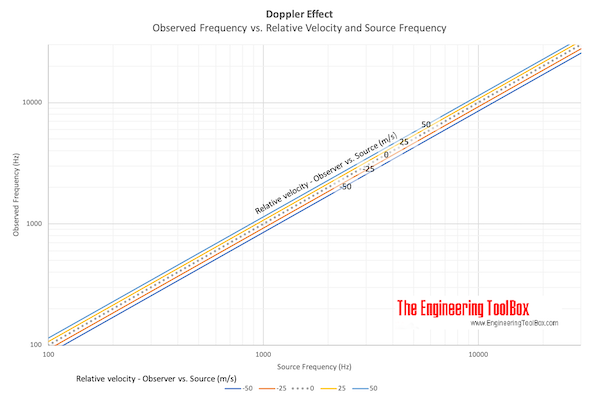Engineering ToolBox - Resources, Tools and Basic Information for Engineering and Design of Technical Applications!

Sound - Doppler Effect

The doppler effect is the change in sound frequency due to the relative motion between a source and a listener.

The change in frequency of sound due to relative motion between a source and a listener is called the Doppler Effect.

The observed sound frequency for a listener can be expressed as

fr = fs (c + vr) / (c + vs)                                 (1)

where

fr = frequency observed by the receiver (1/s, Hz)

fs = frequency emitted from the source (1/s, Hz)

c = speed of sound (m/s)

vr = velocity of the receiver relative to the medium - positive if the receiver is moving towards the source (m/s)

vs = velocity of the source relative to the medium - positive if the source is moving away from the receiver (m/s)

Example - A Train passing a Bell

A train with speed 200 km/h (55.6 m/s) passes a bell with sound frequency 1000 Hz. With an air temperature of 20 oC the speed of sound is 343 m/s.

The frequency observed by a passenger in the train before passing the bell can be calculated as

fr = (1000 Hz) ((343 m/s) + (55.6 m/s)) / ((343 m/s) + (0 m/s))

= 1162 Hz

The frequency observed by the passenger in the train after passing the bell can be calculated as

fr = (1000 Hz) ((343 m/s) + (- 55.6 m/s)) / ((343 m/s) + (0 m/s))

= 838 Hz

Doppler Effect Calculator

Calculate the observed frequency with the calculator below:

fs - frequency emitted from the source (Hz)

c - speed of sound (m/s)

vr - velocity of the receiver relative to the medium (m/s) - m/s vs. km/h vs. mph

vs - velocity of the source relative to the medium (m/s)Download and print the Doppler Effect Chart

Related Topics

• Acoustics - Room acoustics and acoustic properties - decibel A, B and C - Noise Rating (NR) curves, sound transmission, sound pressure, sound intensity and sound attenuation

Engineering ToolBox - SketchUp Extension - Online 3D modeling!

Add standard and customized parametric components - like flange beams, lumbers, piping, stairs and more - to your Sketchup model with the Engineering ToolBox - SketchUp Extension - enabled for use with the amazing, fun and free SketchUp Make and SketchUp Pro .Add the Engineering ToolBox extension to your SketchUp from the SketchUp Pro Sketchup Extension Warehouse!

Translate

Privacy

We don't collect information from our users. Only emails and answers are saved in our archive. Cookies are only used in the browser to improve user experience.

Some of our calculators and applications let you save application data to your local computer. These applications will - due to browser restrictions - send data between your browser and our server. We don't save this data.

Citation

• Engineering ToolBox, (2012). Sound - Doppler Effect. [online] Available at: https://www.engineeringtoolbox.com/doppler-effect-d_1839.html [Accessed Day Mo. Year].

Modify access date.

. .

Scientific Online Calculator1 14